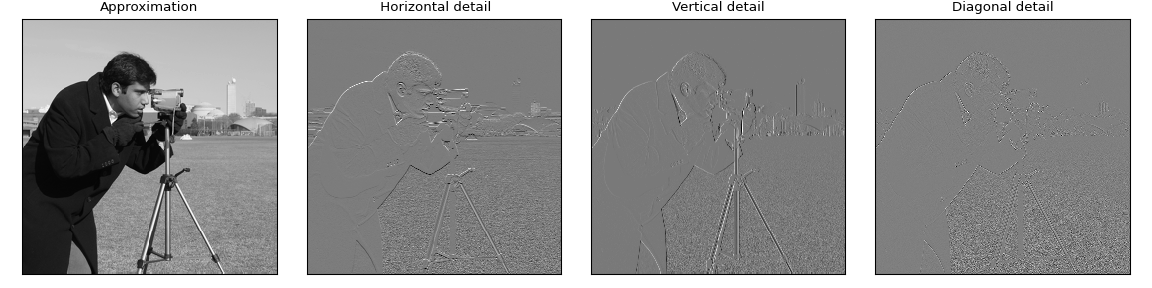# PyWavelets - Wavelet Transforms in Python¶

PyWavelets is open source wavelet transform software for Python. It combines a simple high level interface with low level C and Cython performance.

PyWavelets is very easy to use and get started with. Just install the package, open the Python interactive shell and type:

>>> import pywt
>>> cA, cD = pywt.dwt([1, 2, 3, 4], 'db1')


Voilà! Computing wavelet transforms has never been so simple :)

Here is a slightly more involved example of applying a digital wavelet transform to an image:

import numpy as np
import matplotlib.pyplot as plt

import pywt
import pywt.data

original = pywt.data.camera()

# Wavelet transform of image, and plot approximation and details
titles = ['Approximation', ' Horizontal detail',
'Vertical detail', 'Diagonal detail']
coeffs2 = pywt.dwt2(original, 'bior1.3')
LL, (LH, HL, HH) = coeffs2
fig = plt.figure(figsize=(12, 3))
for i, a in enumerate([LL, LH, HL, HH]):
ax = fig.add_subplot(1, 4, i + 1)
ax.imshow(a, interpolation="nearest", cmap=plt.cm.gray)
ax.set_title(titles[i], fontsize=10)
ax.set_xticks([])
ax.set_yticks([])

fig.tight_layout()
plt.show()## Main features¶

The main features of PyWavelets are:

• 1D, 2D and nD Forward and Inverse Discrete Wavelet Transform (DWT and IDWT)
• 1D, 2D and nD Multilevel DWT and IDWT
• 1D, 2D and nD Stationary Wavelet Transform (Undecimated Wavelet Transform)
• 1D and 2D Wavelet Packet decomposition and reconstruction
• 1D Continuous Wavelet Transform
• Computing Approximations of wavelet and scaling functions
• Over 100 built-in wavelet filters and support for custom wavelets
• Single and double precision calculations
• Real and complex calculations
• Results compatible with Matlab Wavelet Toolbox (TM)

## Getting help¶

Use GitHub Issues, StackOverflow, or the PyWavelets discussions group to post your comments or questions.

PyWavelets is a free Open Source software released under the MIT license.

## Citing¶

If you use PyWavelets in a scientific publication, we would appreciate citations of the project via the following JOSS publication:

Gregory R. Lee, Ralf Gommers, Filip Wasilewski, Kai Wohlfahrt, Aaron O’Leary (2019). PyWavelets: A Python package for wavelet analysis. Journal of Open Source Software, 4(36), 1237, https://doi.org/10.21105/joss.01237.Specific releases can also be cited via Zenodo. The DOI below will correspond to the most recent release. DOIs for past versions can be found by following the link in the badge below to Zenodo: